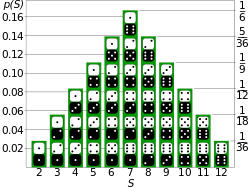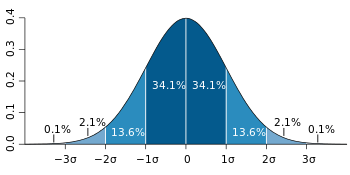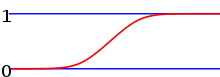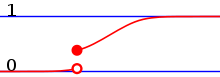# Probability distribution

In probability and statistics, a probability distribution is a mathematical function that, stated in simple terms, can be thought of as providing the probability of occurrence of different possible outcomes in an experiment. For instance, if the random variable X is used to denote the outcome of a coin toss ('the experiment'), then the probability distribution of X would take the value 0.5 for, and 0.5 for.

In more technical terms, the probability distribution is a description of a random phenomenon in terms of the probabilities of events. Examples of random phenomena can include the results of an experiment or survey. A probability distribution is defined in terms of an underlying sample space, which is the set of all possible outcomes of the random phenomenon being observed. The sample space may be the set of real numbers or a higher-dimensional vector space, or it may be a list of non-numerical values; for example, the sample space of a coin flip would be.

Probability distributions are generally divided into two classes. A discrete probability distribution (applicable to the scenario where the set of possible outcomes is discrete, such as in a coin toss or a flip of a dice) can be encoded by a discrete list of the probabilities of the outcomes, known as a probability mass function. On the other hand, a continuous probability distribution (applicable to the scenarios where the set of possible outcomes can take on values in a continuous range (e.g., real numbers), such as the temperature on a given day) is typically described by probability density functions (with the probability of any individual outcome actually being 0). The normal distribution represents a commonly encountered continuous probability distribution. More complex experiments, such as those involving stochastic processes defined in continuous time, may demand the use of more general probability measures.

A probability distribution whose sample space is the set of real numbers is called univariate, while a distribution whose sample space is a vector space is called multivariate. A univariate distribution gives the probabilities of a single random variable taking on various alternative values; a multivariate distribution (a joint probability distribution) gives the probabilities of a random vector—a list of two or more random variables—taking on various combinations of values. Important and commonly encountered univariate probability distributions include the binomial distribution, the hypergeometric distribution, and the normal distribution. The multivariate normal distribution is a commonly encountered multivariate distribution.

## IntroductionThe probability mass function (pmf) p(S) specifies the probability distribution for the sum S of counts from two dice. For example, the figure shows that p(11) = 1/18. The pmf allows the computation of probabilities of events such as P(S > 9) = 1/12 + 1/18 + 1/36 = 1/6, and all other probabilities in the distribution.

To define probability distributions for the simplest cases, one needs to distinguish between discrete and continuous random variables. In the discrete case, it is sufficient to specify a probability mass function assigning a probability to each possible outcome: for example, when throwing a fair dice, each of the six values 1 to 6 has the probability 1/6. The probability of an event is then defined to be the sum of the probabilities of the outcomes that satisfy the event; for example, the probability of the event "the die rolls an even value" isIn contrast, when a random variable takes values from a continuum then typically, any individual outcome has probability zero and only events that include infinitely many outcomes, such as intervals, can have positive probability. For example, the probability that a given object weighs exactly 500 g is zero, because the probability of measuring exactly 500 g tends to zero as the accuracy of our measuring instruments increases. Nevertheless, in quality control one might demand that the probability of a "500 g" package containing between 490 g and 510 g should be no less than 98%, and this demand is less sensitive to the accuracy of our instruments.

Continuous probability distributions can be described in several ways. The probability density function describes the infinitesimal probability of any given value, and the probability that the outcome lies in a given interval can be computed by integrating the probability density function over that interval. On the other hand, the cumulative distribution function describes the probability that the random variable is no larger than a given value; the probability that the outcome lies in a given interval can be computed by taking the difference between the values of the cumulative distribution function at the endpoints of the interval. The cumulative distribution function is the antiderivative of the probability density function provided that the latter function exists.The probability density function (pdf) of the normal distribution, also called Gaussian or "bell curve", the most important continuous random distribution. As notated on the figure, the probabilities of intervals of values correspond to the area under the curve.

## Terminology

As probability theory is used in quite diverse applications, terminology is not uniform and sometimes confusing. The following terms are used for non-cumulative probability distribution functions:

The following terms are somewhat ambiguous as they can refer to non-cumulative or cumulative distributions, depending on authors' preferences:

• Probability distribution function: continuous or discrete, non-cumulative or cumulative.
• Probability function: even more ambiguous, can mean any of the above or other things.

Finally,

• Probability distribution: sometimes the same as probability distribution function, but usually refers to the more complete assignment of probabilities to all measurable subsets of outcomes (i.e. the corresponding probability measure), not just to specific outcomes or ranges of outcomes.

### Basic terms

• Mode: for a discrete random variable, the value with highest probability (the location at which the probability mass function has its peak); for a continuous random variable, the location at which the probability density function has its peak.
• Support: the smallest closed set whose complement has probability zero.
• Head: the range of values where the pmf or pdf is relatively high.
• Tail: the complement of the head within the support; the large set of values where the pmf or pdf is relatively low.
• Expected value or mean: the weighted average of the possible values, using their probabilities as their weights; or the continuous analog thereof.
• Median: the value such that the set of values less than the median has a probability of one-half.
• Variance: the second moment of the pmf or pdf about the mean; an important measure of the dispersion of the distribution.
• Standard deviation: the square root of the variance, and hence another measure of dispersion.
• Symmetry: a property of some distributions in which the portion of the distribution to the left of a specific value is a mirror image of the portion to its right.
• Skewness: a measure of the extent to which a pmf or pdf "leans" to one side of its mean. The third standardized moment of the distribution.
• Kurtosis: a measure of the "fatness" of the tails of a pmf or pdf. The fourth standardized moment of the distribution.

## Cumulative distribution function

Because a probability distribution Pr on the real line is determined by the probability of a scalar random variable X being in a half-open interval (-∞, x], the probability distribution is completely characterized by its cumulative distribution function:## Discrete probability distributionThe probability mass function of a discrete probability distribution. The probabilities of the singletons {1}, {3}, and {7} are respectively 0.2, 0.5, 0.3. A set not containing any of these points has probability zero.The cdf of a discrete probability distribution, ...... of a continuous probability distribution, ...... of a distribution which has both a continuous part and a discrete part.

A discrete probability distribution is a probability distribution characterized by a probability mass function. Thus, the distribution of a random variable X is discrete, and X is called a discrete random variable, ifas u runs through the set of all possible values of X. A discrete random variable can assume only a finite or countably infinite number of values. For the number of potential values to be countably infinite, even though their probabilities sum to 1, the probabilities have to decline to zero fast enough. For example, iffor n = 1, 2, ..., we have the sum of probabilities 1/2 + 1/4 + 1/8 + ... = 1.

Well-known discrete probability distributions used in statistical modeling include the Poisson distribution, the Bernoulli distribution, the binomial distribution, the geometric distribution, and the negative binomial distribution. Additionally, the discrete uniform distribution is commonly used in computer programs that make equal-probability random selections between a number of choices.

### Measure theoretic formulation

A measurable functionbetween a probability spaceand a measurable spaceis called a discrete random variable provided its image is a countable set and the pre-image of singleton sets are measurable, i.e.,for all. The latter requirement induces a probability mass functionvia. Since the pre-images of disjoint sets are disjointThis recovers the definition given above.

### Cumulative density

Equivalently to the above, a discrete random variable can be defined as a random variable whose cumulative distribution function (cdf) increases only by jump discontinuities—that is, its cdf increases only where it "jumps" to a higher value, and is constant between those jumps. The points where jumps occur are precisely the values which the random variable may take.

### Delta-function representation

Consequently, a discrete probability distribution is often represented as a generalized probability density function involving Dirac delta functions, which substantially unifies the treatment of continuous and discrete distributions. This is especially useful when dealing with probability distributions involving both a continuous and a discrete part.

### Indicator-function representation

For a discrete random variable X, let u0, u1, ... be the values it can take with non-zero probability. DenoteThese are disjoint sets, and by formula (1)It follows that the probability that X takes any value except for u0, u1, ... is zero, and thus one can write X asexcept on a set of probability zero, whereis the indicator function of A. This may serve as an alternative definition of discrete random variables.

## Continuous probability distribution

A continuous probability distribution is a probability distribution that has a cumulative distribution function that is continuous. Most often they are generated by having a probability density function. Mathematicians call distributions with probability density functions absolutely continuous, since their cumulative distribution function is absolutely continuous with respect to the Lebesgue measure λ. If the distribution of X is continuous, then X is called a continuous random variable. There are many examples of continuous probability distributions: normal, uniform, chi-squared, and others.

Intuitively, a continuous random variable is the one which can take a continuous range of values—as opposed to a discrete distribution, where the set of possible values for the random variable is at most countable. While for a discrete distribution an event with probability zero is impossible (e.g., rolling 31/2 on a standard dice is impossible, and has probability zero), this is not so in the case of a continuous random variable. For example, if one measures the width of an oak leaf, the result of 3½ cm is possible; however, it has probability zero because uncountably many other potential values exist even between 3 cm and 4 cm. Each of these individual outcomes has probability zero, yet the probability that the outcome will fall into the interval (3 cm, 4 cm) is nonzero. This apparent paradox is resolved by the fact that the probability that X attains some value within an infinite set, such as an interval, cannot be found by naively adding the probabilities for individual values. Formally, each value has an infinitesimally small probability, which statistically is equivalent to zero.

Formally, if X is a continuous random variable, then it has a probability density function ƒ(x), and therefore its probability of falling into a given interval, say [a, b] is given by the integralIn particular, the probability for X to take any single value a (that is aXa) is zero, because an integral with coinciding upper and lower limits is always equal to zero.

The definition states that a continuous probability distribution must possess a density, or equivalently, its cumulative distribution function be absolutely continuous. This requirement is stronger than simple continuity of the cumulative distribution function, and there is a special class of distributions, singular distributions, which are neither continuous nor discrete nor a mixture of those. An example is given by the Cantor distribution. Such singular distributions however are never encountered in practice.

Note on terminology: some authors use the term "continuous distribution" to denote the distribution with continuous cumulative distribution function. Thus, their definition includes both the (absolutely) continuous and singular distributions.

By one convention, a probability distributionis called continuous if its cumulative distribution functionis continuous and, therefore, the probability measure of singletonsfor all.

Another convention reserves the term continuous probability distribution for absolutely continuous distributions. These distributions can be characterized by a probability density function: a non-negative Lebesgue integrable functiondefined on the real numbers such thatDiscrete distributions and some continuous distributions (like the Cantor distribution) do not admit such a density.

## Some properties

• The probability distribution of the sum of two independent random variables is the convolution of each of their distributions.
• Probability distributions are not a vector space—they are not closed under linear combinations, as these do not preserve non-negativity or total integral 1—but they are closed under convex combination, thus forming a convex subset of the space of functions (or measures).

## Kolmogorov definition

In the measure-theoretic formalization of probability theory, a random variable is defined as a measurable function X from a probability spaceto measurable space. A probability distribution of X is the pushforward measure X*P  of X , which is a probability measure onsatisfying X*P = PX −1.

## Random number generation

A frequent problem in statistical simulations (the Monte Carlo method) is the generation of pseudo-random numbers that are distributed in a given way. Most algorithms are based on a pseudorandom number generator that produces numbers X that are uniformly distributed in the interval [0,1]. These random variates X are then transformed via some algorithm to create a new random variate having the required probability distribution.

## Applications

The concept of the probability distribution and the random variables which they describe underlies the mathematical discipline of probability theory, and the science of statistics. There is spread or variability in almost any value that can be measured in a population (e.g. height of people, durability of a metal, sales growth, traffic flow, etc.); almost all measurements are made with some intrinsic error; in physics many processes are described probabilistically,from the kinetic properties of gases to the quantum mechanical description of fundamental particles. For these and many other reasons, simple numbers are often inadequate for describing a quantity, while probability distributions are often more appropriate.

As a more specific example of an application, the cache language models and other statistical language models used in natural language processing to assign probabilities to the occurrence of particular words and word sequences do so by means of probability distributions.

## Common probability distributions

The following is a list of some of the most common probability distributions, grouped by the type of process that they are related to. For a more complete list, see list of probability distributions, which groups by the nature of the outcome being considered (discrete, continuous, multivariate, etc.)

Note also that all of the univariate distributions below are singly peaked; that is, it is assumed that the values cluster around a single point. In practice, actually observed quantities may cluster around multiple values. Such quantities can be modeled using a mixture distribution.

• Normal distribution (Gaussian distribution), for a single such quantity; the most common continuous distribution
• Rayleigh distribution, for the distribution of vector magnitudes with Gaussian distributed orthogonal components. Rayleigh distributions are found in RF signals with Gaussian real and imaginary components.
• Rice distribution, a generalization of the Rayleigh distributions for where there is a stationary background signal component. Found in Rician fading of radio signals due to multipath propagation and in MR images with noise corruption on non-zero NMR signals.

### Useful as conjugate prior distributions in Bayesian inference

Main article: Conjugate priorWikimedia Commons has media related to Probability distribution.